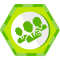# 2.C++下的资源管理方式

## 2.2 通过智能指针进行资源管理

### 2.2.1 unique_ptr

unique_ptr负责独占对应对象的所有权，一旦unique_ptr析构，那么对应对象自动销毁，unique_ptr对应对象的控制权可以转移，但不可拷贝，具有独占性。可通过实例查看。

``````class A
{
public:
A()
{
std::cout << "A construct." << std::endl;
a = 10;
b = 11;
}

~A()
{
std::cout << "A destruct." << std::endl;
}

void func()
{
std::cout << "A::func()" << std::endl;
std::cout << a << " " << b << std::endl;
}

private :
int a;
int b;
};

void unique_ptr_test()
{
{
std::unique_ptr<A> up1(new A());
up1->func();

{
std::unique_ptr<A> up2(std::move(up1));
std::cout << "control from up1 to up2." << std::endl;
up2->func();
//up1->func();  此处执行会报错，up1为empty
up1 = std::move(up2);
std::cout << "return control from up2 to up1" << std::endl;
up1->func();
}

}

{
A* as = new A;
std::unique_ptr<A[]> pas(as);
}
}
``````
``````A construct.
A::func()
10 11
control from up1 to up2.
A::func()
10 11
return control from up2 to up1
A::func()
10 11
A destruct.
A construct.
A construct.
A construct.
A construct.
A construct.
A destruct.
A destruct.
A destruct.
A destruct.
A destruct.
``````

1. unique_ptr对对象的独占性，可以避免忘记delete而导致的资源泄漏。
2. unique_ptr的控制转可以转移，一旦转移就变成empty.
3. 不能由多个unique_ptr一起控制单个对象。
4. unique_ptr可以管理对象数组，并保证多个对象的正确释放

### 2.2.2 shared_ptr 和 weak_ptr

shared_ptr和weak_ptr是一对组合。shared_ptr是计数型智能指针，属于强引用，每一个关联都有计数，而weak_ptr是弱引用，不会影响计数功能。

shared_ptr独立测试

``````void shared_ptr_test()
{
std::shared_ptr<A>  sp1(new A());
std::cout << sp1.use_count() << std::endl;

{
std::shared_ptr<A> sp2(sp1);
std::cout << sp2.use_count() << std::endl;
std::shared_ptr<A> sp3(sp2);
std::cout << sp3.use_count() << std::endl;
{
std::shared_ptr<A> sp4(sp3);
std::cout << sp4.use_count() << std::endl;

}
}
}

A construct.
1
2
3
4
A destruct.
``````

weak_ptr独立测试 在微软 MSDN上看到如下一段话：

``````The template class describes an object that points to a resource that is managed by one or more shared_ptr Class objects.

The weak_ptr objects that point to a resource do not affect the resource's reference count.
weak_ptr对象指向一个资源，不会影响该资源的引用计数。

Thus, when the last shared_ptr object that manages that resource is destroyed the resource will be freed, even if there  are weak_ptr objects pointing to that resource. This is essential for avoiding cycles in data structures.

void weak_ptr_test()
{
{
std::shared_ptr<A> sp1(new A());
std::weak_ptr<A> wp1(sp1);
std::cout << "shared use count: " << sp1.use_count() << std::endl;
std::cout << "weak_ptr use count: " << wp1.use_count() << std::endl;

{
std::shared_ptr<A> sp2(new A());
std::weak_ptr<A> wp2(sp1);
std::cout << "shared use count: " << sp2.use_count() << std::endl;
std::cout << "weak_ptr use count: " << wp2.use_count() << std::endl;
}
}
}

A construct.
shared use count: 1
weak_ptr use count: 1
A construct.
shared use count: 1
weak_ptr use count: 1
A destruct.
A destruct.

``````

weak_ptr的一个作用是可以有效判断某个对象是否还存活，示例如下：

``````void shared_weak_ptr_test()
{
std::weak_ptr<A> wp1;
{
std::shared_ptr<A> sp1(new A());
wp1 = sp1;
std::cout << "shared use count: " << sp1.use_count() << std::endl;
std::cout << "weak_ptr use count: " << wp1.use_count() << std::endl;
}

if (wp1.lock() != nullptr)
{
std::cout << "resource exists." << std::endl;
}
else
{
std::cout << "resource not exists." << std::endl;
}

}

A construct.
shared use count: 1
weak_ptr use count: 1
A destruct.
resource not exists.
``````

shared_ptr和weak_ptr联合测试 为什么shared_ptr和weak_ptr总是联合使用呢，在陈硕《Linux多线程服务端编程》中用了一个非常生动的示例进行说明，就是观察者模式。当被观察者发生某个事件，需要通知多个观察者时，往往是通过指针依次调用。这边存在的一个问题就是，如果某个观察者在其他线程中被删除，所指对象已经被删除，那么在调用方法时，就会出现问题。因为对象已经无效。我自己编写了一个简易示例，并未在多线程中运行，但是可以作为说明。

``````class Observer
{
public:
Observer(int32_t id) : observer_id(id)
{}

void update()
{
std::cout << observer_id <<  " Observer " << std::endl;
}

private:
int32_t observer_id;
};

class Observable
{
public:
void notifyall()
{
std::lock_guard<std::mutex> guard(observable_mutex);

for(std::vector<std::weak_ptr<Observer>>::iterator it = observers.begin(); it != observers.end(); )
{
std::weak_ptr<Observer> ov = *it;
std::shared_ptr<Observer> sp(ov.lock());
if (sp != nullptr)
{
sp->update();
it++;
}
else
{
it = observers.erase(it);
}
}

if (observers.size() == 0)
{
std::cout << "no observers. ." << std::endl;
}
}

void reg(std::weak_ptr<Observer> ob)
{
std::lock_guard<std::mutex> guard(observable_mutex);
observers.push_back(ob);
}

private:
std::vector<std::weak_ptr<Observer>> observers;
std::mutex  observable_mutex;
};

void observer_test()
{
std::shared_ptr<Observable> bk;
{
std::shared_ptr<Observer> ob1(new Observer(1));
std::shared_ptr<Observer> ob2(new Observer(2));
std::shared_ptr<Observer> ob3(new Observer(2));

std::shared_ptr<Observable> obed1(new Observable);
obed1->reg(ob1);
obed1->reg(ob2);
obed1->reg(ob3);

obed1->notifyall();

bk = obed1;
}

bk->notifyall();

}

1 Observer
2 Observer
2 Observer
no observers. .

``````

### 2.2.3 auto_ptr

cpp还有其他类型的智能指针，比如auto_ptr,不过目前在c++11中并不被推荐使用。 auto_ptr和unique_ptr有些类似，都是表达对资源的唯一所有权，但是区别是，auto_ptr可以通过赋值操作默认转移所有权，而unique_ptr需要显式的表达转移动作。

``````void auto_ptr_test()
{
{
std::auto_ptr<A> ap(new A());
ap->func();

std::auto_ptr<A> ap2 = ap;
ap2->func();
ap->func();  //此处会出现错误，因为ap已经不拥有A对象的资源，在访问对象内部变量的时候，自然会报错
}
}
``````

``````	{
A* a = new A();
std::auto_ptr<A> ap(a);
std::auto_ptr<A> ap2(a);
}
``````

## 2.3 C++下的垃圾回收器

blink中的垃圾回收器 从一般性考虑来讲，Cpp可以很精确的控制内存，也有各种智能指针使用，为什么还要有垃圾回收呢。这不是剥夺了Cpp程序员DEBUG的乐趣么。文章解释说因为工程规模大，即使有智能指针等各种技术，还是避免不了内存泄漏等问题，最后还是在相应项目下提供一套通用的垃圾回收机制。

blink github链接

# 3. JAVA下的资源管理（回收）方式

JAVA下的垃圾回收并没有采用计数法。因为计数无法解决循环引用的问题。思考下C++中相互引用的两个类，都拥有对方的shared_ptr类型的指针，如何正确释放对象。这也是为啥有weak_ptr的原因。

JAVA中所有通过new出来的资源对象存放在堆中，相关的引用放在堆栈上。在垃圾回收时，采取可达性分析。通过一系列的“GCRoots”对象作为起点进行搜索，所有可直接或者间接与GC Roots相连的为有效对象，其他则为无效对象；区分开有效、无效对象后，就可进一步处理。

## 3.1 标记清除算法（mark and sweep）

1. 标记阶段，通过从GC Roots进行搜索，区分开所有可用对象及无效对象；
2. 清除阶段，将所有无效对象串入到空闲空间链表中，用于后续对象的空间分配。

# 3.4 分代收集算法（Generational Collection）© 著作权归作者所有### 西昆仑Java finalize方法

《JAVA编程思想》： java提供finalize()方法，垃圾回收器准备释放内存的时候，会先调用finalize()。 (1).对象不一定会被回收。 (2).垃圾回收不是析构函数。 (3).垃圾回收只与内存有关。 (4)....

2017/10/22
35
0

Java区别于C++ 表面看来两者最大的不同在于Java没有指针，或者说，Java满地都是指针。对于编程者而言Java的这种设计是安全且更易用的。说Java满地是指针的原因在于它把指针的功能隐藏了，其实...

Ace☞Tseng
2012/10/09
313
0

2013/01/06
1K
1
Java程序员如何高效而优雅地入门C++

Java程序员如何高效而优雅地入门Cpp，由于工作需要，需要用C++写一些模块。关于C++ 的知识结构，虽说我有过快速学习很多新语言的经验，但对于C++ 我也算是老手，但也还需要心生敬畏，本文会从...

2018/04/23
82
1

C语言 虽说C语言在内存管理方面存在严重的缺陷，不过它还是在某些应用领域里称王称霸。对于那些要求最高的效率，良好的实时性，或者与操作系统内核紧密关联的程序来说，C仍然是很好的选择。 ...

kouxunli1
2015/01/07
273
0

Giraph源码分析（八）—— 统计每个SuperStep中参与计算的顶点数目

4
0
Xss过滤器（Java）

10
0
Navicat 快捷键

10
0
Set 和 Map

Set 1：基本概念 类数组对象, 内部元素唯一 let set = new Set([1, 2, 3, 2, 1]); console.log(set); // Set(3){ 1, 2, 3 } [...set]; // [1, 2, 3] 接收数组或迭代器对象 ...

4
0
PyTorch入门笔记一

6
0